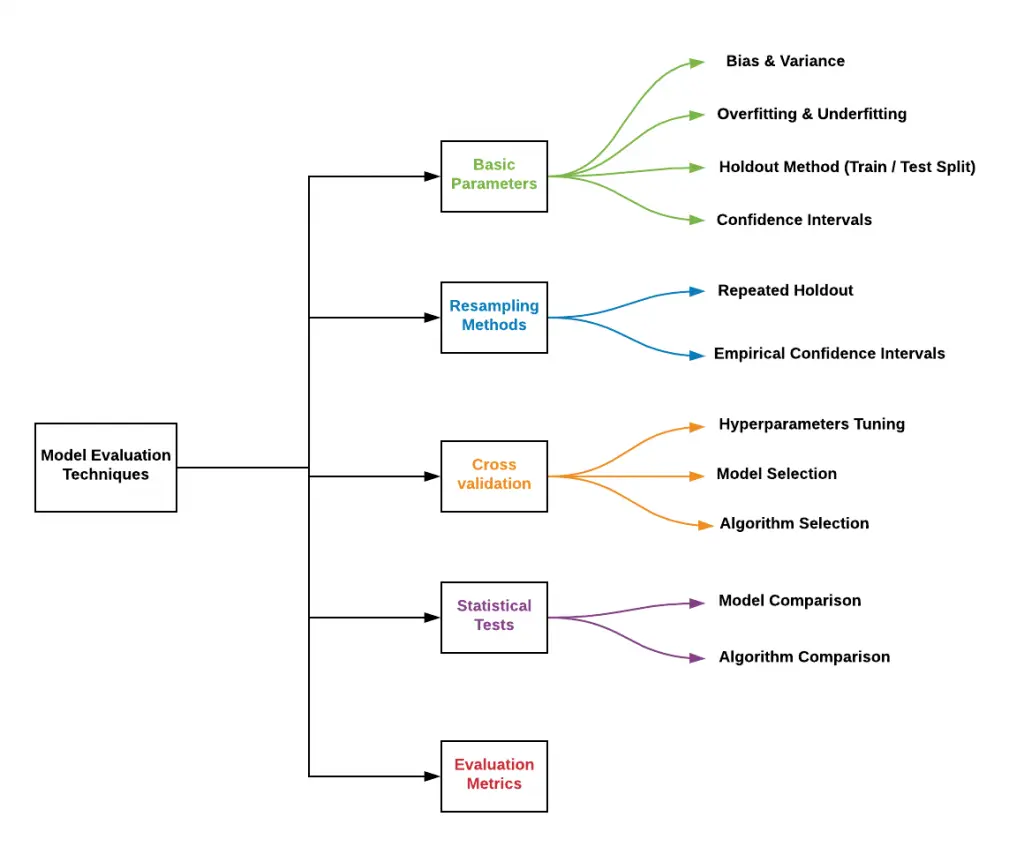# Model Evaluation

Model evaluation is a critical process in machine learning that is used to assess the performance of a trained model. It involves comparing the predicted values from the model to the actual values in the test dataset to determine the level of agreement between the two.

Some key terms and concepts related to model evaluation include:

• Accuracy: a metric that measures the percentage of correct predictions made by the model

• Precision: a metric that measures the percentage of true positives out of all predicted positives

• Recall: a metric that measures the percentage of true positives out of all actual positives

• F1 score: a metric that combines precision and recall into a single score

• Area Under the Curve (AUC): a metric that measures the performance of a binary classification model by calculating the area under the Receiver Operating Characteristic (ROC) curve

• Overfitting: a common problem in model evaluation where the model is too complex and performs well on the training data but poorly on new, unseen data

• Underfitting: a common problem in model evaluation where the model is too simple and performs poorly on both the training data and new, unseen data

• Cross-validation: a technique used to assess the performance of a model by splitting the data into multiple subsets and training and evaluating the model on each subset

• Regularization: a technique used to prevent overfitting by adding a penalty term to the loss function that discourages the model from being too complex.(Image source: Data Analytics)

## Examples of model evaluation:

• Classification: In a binary classification problem, model evaluation can be performed by calculating metrics such as accuracy, precision, recall, F1 score, and AUC. For example, in a medical diagnosis problem, a binary classification model can be trained to predict whether a patient has a certain disease or not. The model can then be evaluated on a test dataset to assess its performance in terms of accuracy, precision, recall, F1 score, and AUC.

• Regression: In a regression problem, model evaluation can be performed by calculating metrics such as mean absolute error, mean squared error, and R-squared. For example, in a real estate price prediction problem, a regression model can be trained to predict the price of a house based on features such as its size, location, and number of bedrooms. The model can then be evaluated on a test dataset to assess its performance in terms of mean absolute error, mean squared error, and R-squared.

• Image classification: In an image classification problem, model evaluation can be performed by calculating metrics such as top-1 accuracy and top-5 accuracy. For example, in an image recognition problem, a model can be trained to classify images into different categories, such as cats and dogs. The model can then be evaluated on a test dataset to assess its performance in terms of top-1 accuracy (the percentage of images that are correctly classified as the top predicted category) and top-5 accuracy (the percentage of images that are correctly classified as one of the top 5 predicted categories).

Overall, model evaluation is a critical step in the machine learning workflow, as it helps to ensure that the model is accurate and reliable for real-world use cases. By understanding key terms and concepts related to model evaluation, developers can better assess the performance of their models and make improvements as needed.